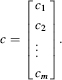Appendix A

BASIC MATRIX ALGEBRA FOR ECONOMETRICS

A.1 MATRIX DEFINITIONS

A.1.a Definitions

An m × n matrix is a rectangular array of elements arranged in m rows and n columns. A general layout of a matrix is given byIn this general form, we can easily index any element of the matrix. For instance, the element in the ith row and jth column is given by aij. It is straightforward to create matrices in Proc IML. For example, the Proc IML command A = {2 4,3 1} will create the 2 × 2 matrixA row vector of order n is a matrix with one row and n columns. The general form of a row vector is y = [y1 y2yn.] A column vector of order m is a matrix with m rows and one column. The general form of a column vector isIt is straightforward to create row and column vectors in Proc IML. For example, the Proc IML command y = {2 4} will create the row vector y = [2 4], while the Proc IML command c={–3, 4} will create the column vectorOf course, these definitions can easily be extended to matrices of any desired dimension and consequently the Proc IML code can be adjusted to accommodate these changes. ...

Get Applied Econometrics Using the SAS® System now with the O’Reilly learning platform.

O’Reilly members experience live online training, plus books, videos, and digital content from nearly 200 publishers.